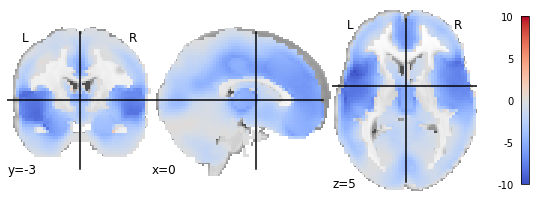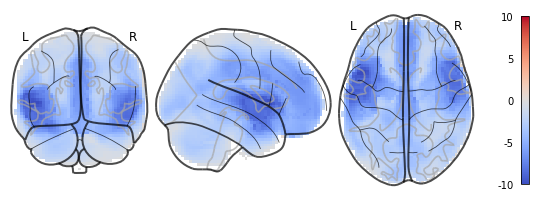# Make nilearn.plotting.plot_stat_map and nilearn.plotting.plot_glass_brain plotting the same image except for the background image

I would like to plot my statistical image using `nilearn.plotting.plot_glass_brain`. Two notes that might be related to my question:

First of all, my image is not in MNI152 space, which according to the documentation of `nilearn.plotting.plot_glass_brain` should be the case in order to “to work properly”.
Secondly, my statistical image is restricted to grey matter area only, so the output plot should handle this.

When I use `nilearn.plotting.plot_stat_map`, everything works as expected. White matter areas in the center are ‘left blank’:However, when I use `nilearn.plotting.plot_glass_brain`, I get the following output:This is, of course, misleading, as it suggests that there are values in white matter areas, where there aren’t any.

In general, I also noted that I had to change and add some keyword arguments to `nilearn.plotting.plot_glass_brain` in order to give similar results to `nilearn.plotting.plot_stat_map`, which made me wonder, why the two functions differ so much in their default settings when naively spoken, the only difference between the two is the background image?

Here’s my code:

``````# plot statistical image
plotting.plot_stat_map(csz_unthresholded,
colorbar=True,
symmetric_cbar=True,
cmap='coolwarm',
cut_coords=[0,-3,5],
threshold=1e-6,
)

# plot statistical image
plotting.plot_glass_brain(csz_unthresholded,
colorbar=True,
symmetric_cbar=True,
cmap='coolwarm',
cut_coords=[0,-3,5],
threshold=1e-6,
vmax=10,
plot_abs=False)
``````

the difference is not the background image. plot_stat_map plots slices, plot_glass_brain plots projections

This is called “maximum intensity projection”: along a direction, you plot the maximal value. This is useful only when the image you plot is sparse.
HTH,
Bertrand

Thanks for the explanation. The difference between ‘projection’ and ‘maximum intensity projection’ made it clear for me.

Note, that the term ‘maximum intensity projection’ appears in this part of the documentation, but not in the beginning the documentation of the function itself (only when you scroll down until you find the documentation for `plot_abs`). Maybe it would be useful, if this would also appear in the beginning. Also, if I correctly get the concept for ‘maximum intensity projection’ it wouldn’t make sense for this function to accept `cut_coords`, right? Since it will always plot the maximum value?